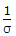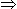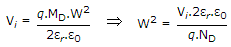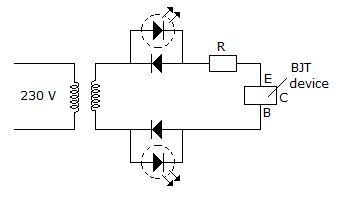Courses

# Test: Electronic Devices And Circuits - 2

## 25 Questions MCQ Test Electronic Devices | Test: Electronic Devices And Circuits - 2

Description
This mock test of Test: Electronic Devices And Circuits - 2 for Electrical Engineering (EE) helps you for every Electrical Engineering (EE) entrance exam. This contains 25 Multiple Choice Questions for Electrical Engineering (EE) Test: Electronic Devices And Circuits - 2 (mcq) to study with solutions a complete question bank. The solved questions answers in this Test: Electronic Devices And Circuits - 2 quiz give you a good mix of easy questions and tough questions. Electrical Engineering (EE) students definitely take this Test: Electronic Devices And Circuits - 2 exercise for a better result in the exam. You can find other Test: Electronic Devices And Circuits - 2 extra questions, long questions & short questions for Electrical Engineering (EE) on EduRev as well by searching above.
QUESTION: 1

### An incremental model of a solid state device is one which represents the

Solution:

Incremental model is used for ac response at one operating point.

QUESTION: 2

### What is the correct sequence of the following step in the fabrication of a monolithic, Bipolar junction transistor? Emitter diffusionBase diffusionBuried layer formationE pi-layer formation Select the correct answer using the codes given below:

Solution:

It is always non-linear.

QUESTION: 3

### For an n-channel enhancement type MOSFET, if the source is connected at a higher potential than that of the bulk (VSB > 0), the threshold voltage VT of the MOSFET will

Solution:

VT depends upon MOSFET construction, hence it will Independent from MOSFET parameters.

QUESTION: 4

Which of the following is used for generating time varying wave forms?

Solution:

Its output is used to trigger SCR.

QUESTION: 5

Calculate the resistivity of n-type semiconductor from the following data, Density of holes = 5 x 1012 cm-3. Density of electrons = 8 x 1013 cm-3, mobility of conduction electron = 2.3 x 104 cm2/ V-sec and mobility of holes = 100 cm2/V-sec.

Solution:

Resistivity(r) =.σ = e(neue + nnun).

QUESTION: 6

An one sided abrupt junction has 1021/m3 of dopants on the lightly doped side, zero bias voltage and a built-in potential of 0.2 V. The depletion width of abrupt junction.(q = 1.6 x 10-19 C, εr =16, ε0 = 8.87 x 10-12 F/m) is

Solution:.

QUESTION: 7

n-type semiconductors

Solution:

n type semiconductor is produced when pentavalent impurity is added.

QUESTION: 8

In all metals

Solution:

In all metals conductivity decreases (and resistance increases) with increase in temperature.

QUESTION: 9

The voltage across a zener diode

Solution:

Zener diode is always reverse biased.

QUESTION: 10

Assertion (A): Two transistors one n-p-n and the other p-n-p are identical in all respects (doping, construction, shape, size). The n-p-n transistor will have better frequency response.

Reason (R): The electron mobility is higher than hole mobility.

Solution:

Therefore mostly npn transistors are used.

QUESTION: 11

The threshold voltage of an n-channel enhancement mode MOSFET is 0.5 when the device is biased at a gate voltage of 3V. Pinch off would occur at a drain voltage of

Solution:

It is used with reverse bias.

QUESTION: 12

Which of these has degenerate p and n materials?

Solution:

Tunnel diode has heavily doped p and n layers called degenerate p and n materials.

QUESTION: 13

A Schottky diode clamp is used along with switching BJT for

Solution:

Schottky diode has very low switching time.

QUESTION: 14

From the given circuit below, we can conclude that.Solution:

According to figure, both the LED is glowing, which indicate that circuit is complete in both the half cycle of a.c. signal.

Therefore Emitter and Base junction will act as short circuit in both direction, which indicate transistor is faulty.

QUESTION: 15

In a piezoelectric crystal, applications of a mechanical stress would produce

Solution:

In piezoelectric materials mechanical stress produces electric polarization.

QUESTION: 16

In which of the following is the width of junction barrier very small?

Solution:

Schottky diode has very small depletion layer.

QUESTION: 17

If the reverse voltage across a p-n junction is increased three times, the junction capacitance

Solution:

Increase of reverse voltage widens the depletion layer and junction capacitance decreases. However the decrease in capacitance is not proportional to increase in voltage.

QUESTION: 18

Which of these has highly doped p and n region?

Solution:

Tunnel diode has heavily doped p and n regions leading to very thin depletion layer.

QUESTION: 19

Measurement of Hall coefficient enables the determination of

Solution:

If a potential difference is developed across a current carrying metal strip when the strip is placed in transverse magnetic field.

Hall effect is very weak in metals, but it is large semiconductors.

QUESTION: 20

The units for transconductance are

Solution:

Its units are the same as the units of conductance.

QUESTION: 21

The amount of photoelectric emission current depends on the frequency of incident light.

Solution:

It depends on intensity of incident light.

QUESTION: 22

When a p-n junction is forward biased

Solution:

Forward voltage decreases the width of depletion layer leading to low resistance.

QUESTION: 23

The carriers of n channel JFET are

Solution:

In n type semiconductors carriers are electrons.

QUESTION: 24

The depletion layer around p-n junction in JFET consists of

Solution:

Depletion layer always has immobile charges.

QUESTION: 25

Junction temperature is always the same as room temperature.

Solution:

When the device is being used, junction temperature is higher than room temperature.